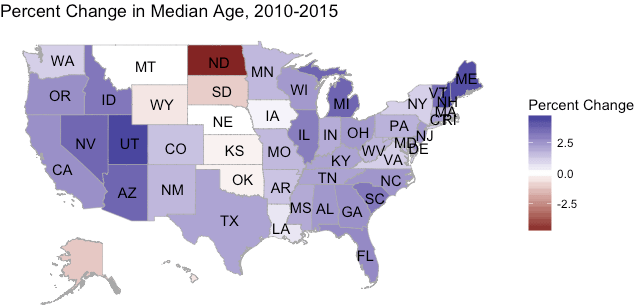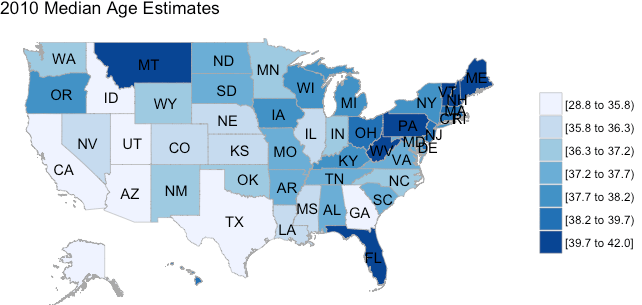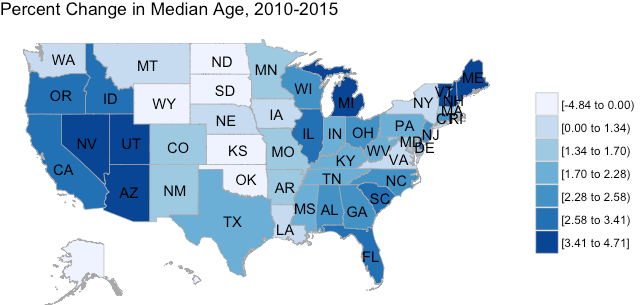# Choroplethr v3.6.1 is now on CRAN

April 24, 2017
By

Want to share your content on R-bloggers? click here if you have a blog, or here if you don't.

Choroplethr version 3.6.1 is now on CRAN. This version makes it easier to

1. Calculate the percent change in demographic datasets
2. Visualize that percent change on a map

As an example, the following map shows how the median age in US States changed between 2010 and 2015:In the above map, both the extreme positive and negative values stand out.

To get this version, simply type the following from an R console:

```install.packages("choroplethr")
packageVersion("choroplethr")
 ‘3.6.1’
```

## New Data

To facilitate demonstrating this functionality I added two new datasets to choroplethr: df_state_age_2010 and df_state_age_2015. You can load them as follows:

```library(choroplethr)

data(df_state_age_2010)
data(df_state_age_2015)
```

You can inspect and map each dataset like this:

```head(df_state_age_2010)
region     value
1 alabama    37.5
3 arizona    35.5
4 arkansas   37.2
5 california 34.9

state_choropleth(df_state_age_2010, title="2010 Median Age Estimates")

```## New Function: calculate_percent_change

To facilitate calculating the percent change between two “choroplethr-style” dataframes I created the function calculate_percent_change.

This function assumes that it is given two dataframes, each with a column called region and value. The dataframes are joined by the region column, and the percent change between their value columns is computed. The result is rounded to two decimal places.

calculate_percent_change itself returns a dataframe with region and value columns. This makes the result easy to map with choroplethr functions.

```df_age_diff = calculate_percent_change(df_state_age_2010, df_state_age_2015)

region     value.x value.y value
1 alabama    37.5    38.4    2.40
3 arizona    35.5    36.8    3.66
4 arkansas   37.2    37.7    1.34
5 california 34.9    35.8    2.58

state_choropleth(df_age_diff, title="Percent Change in Median Age, 2010-2015")
```## New Visualization Option: Divergent Scale

The above map is not as informative as it could be, because the negative values don’t stand out very much. It’s also not clear which regions are near 0 or near -5%.

Choroplethr 3.6.1 solves this problem by letting uses select a divergent scale. To do this, simply set the parameter num_colors = 0.

```state_choropleth(df_age_diff,
title      = "Percent Change in Median Age, 2010-2015",
legend     = "Percent Change",
num_colors = 0)

```With the divergent scale, it’s clear where both the negative and positive outliers are.

## Closing Notes

It has always been possible to use a divergent scale via choroplethr’s object-oriented system. Functions like state_choropleth exist to hide those details. That, in turn, allows beginners to create maps with minimal work. Adding support for divergent scales with the num_colors parameter is another step in that direction.

The only drawback is that the num_colors parameter is now overloaded:

1. num_colors=0 will use a divergent scale. As we’ve seen above, this is useful when the values are both positive and negative.
2. num_colors=1 will use a continuous scale. This is useful for detecting outliers.
3. Setting num_colors to values in [2, 7] will use that many quantiles. This is a useful catch-all for detecting patterns within the data.

At some point it might make sense for me to rethink choroplethr’s API for setting scales. But at this point I wanted to add the new functionality without breaking any existing code.The post Choroplethr v3.6.1 is now on CRAN appeared first on AriLamstein.com.

R-bloggers.com offers daily e-mail updates about R news and tutorials about learning R and many other topics. Click here if you're looking to post or find an R/data-science job.
Want to share your content on R-bloggers? click here if you have a blog, or here if you don't.